# Correlation

The correlation coefficient (a value between -1 and +1) tells you how strongly two variables are related to each other. We can use the CORREL function or the Analysis Toolpak add-in in Excel to find the correlation coefficient between two variables.

- A correlation coefficient of +1 indicates a perfect positive correlation. As variable X increases, variable Y increases. As variable X decreases, variable Y decreases.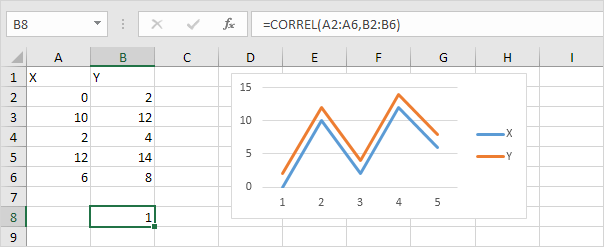- A correlation coefficient of -1 indicates a perfect negative correlation. As variable X increases, variable Z decreases. As variable X decreases, variable Z increases.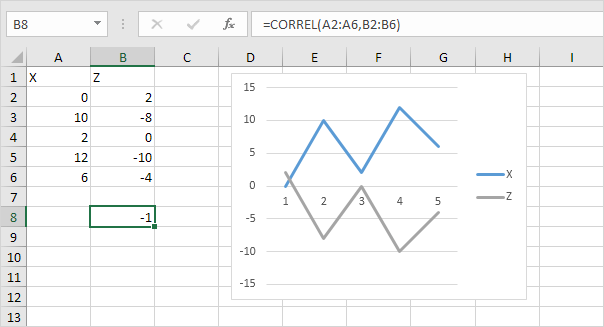- A correlation coefficient near 0 indicates no correlation.

To use the Analysis Toolpak add-in in Excel to quickly generate correlation coefficients between multiple variables, execute the following steps.

1. On the Data tab, in the Analysis group, click Data Analysis.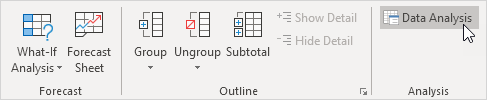2. Select Correlation and click OK.3. For example, select the range A1:C6 as the Input Range.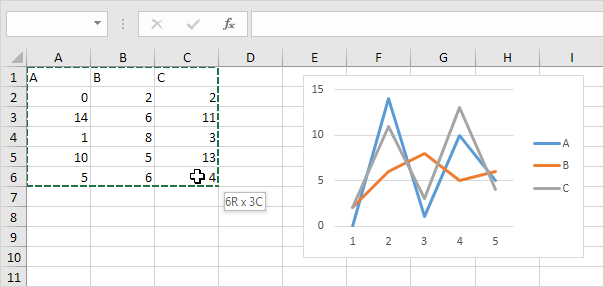4. Check Labels in first row.

5. Select cell A8 as the Output Range.

6. Click OK.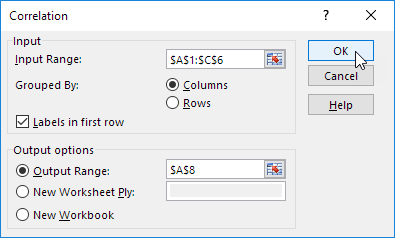Result.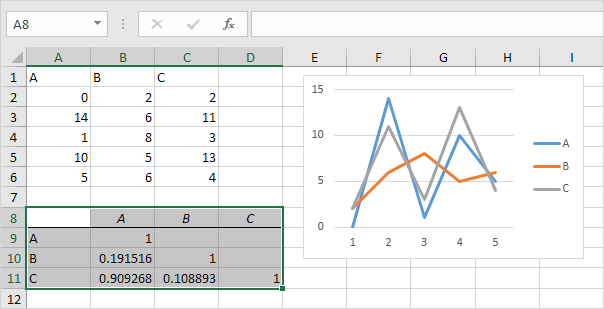Conclusion: variables A and C are positively correlated (0.91). Variables A and B are not correlated (0.19). Variables B and C are also not correlated (0.11) . You can verify these conclusions by looking at the graph.

Go to Next Chapter: Create a Macro